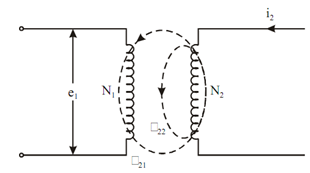## Two Isolated Coils Assignment Help

Assignment Help: >> Inductance - Two Isolated Coils

Two Isolated Coils:

Let current i1 is alternating current and producing flux φ1  (per turn).

Then           φ1  = φ11 + φ12

where φ 11 = flux linked with coil 1 only (leakage flux), and

φ 12 = flux reaches to coil 2 (mutual or useful flux).

Now the emf is induced in both the coils. In coil 1, emf e1 is induced because of its self inductance L1 and in coil 2 emf e2 is induced because of mutual inductance M between coils 1 and 2.

e1  = L1 di1 / dt                      (by Eq. (4))

Likewise, we may write the expression for e2

e2 = M (di1 /dt)

where M = mutual inductance among coils 1 and 2 (in henry)

e2 may also be written as :

e2   = N 2 d φ12 / dt

On equating Eqs. (6) and (7)

M  di1/ dt = N2 (d φ12 /dt)

⇒         M = N2 (d φ12 /di1)

Assuming the zero initial conditions

M = N212/ i2)

Now consider the reverse case, in which we give the supply to the secondary coil and finding the induced emf in primary coil.Figure: Two Isolated Coils with Current i2

φ2  = φ21 + φ22

Where φ 21 = mutual flux,

φ 22  = leakage flux, and

φ 2 = total flux produced by i2.

So the induced emf

e2 = L2  ( di2 /dt)

(because of self inductance L2 of the coil).